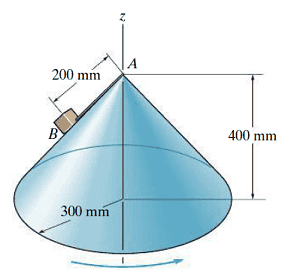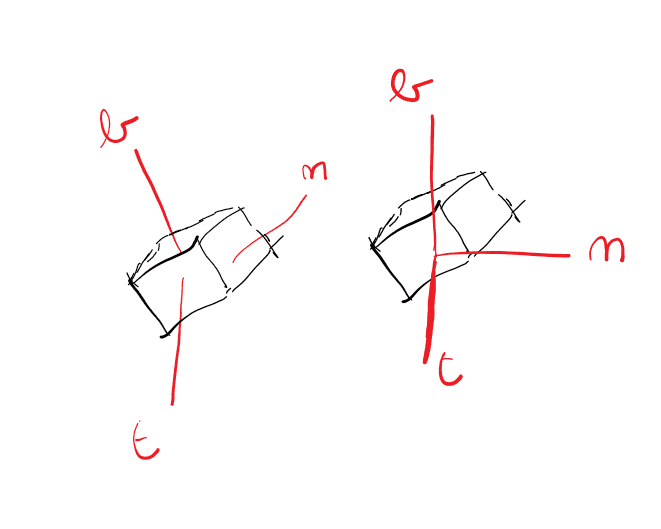# Circular motion along cone

Homework Statement
The block B , having a mass of 0.2 kg, is attached to the vertex A of the right circular cone using a light cord. The cone is rotating at a constant angular rate about the z axis such that the block attains a speed of 0.5 m/s.
Part A
At this speed, determine the tension in the cord. Neglect the size of the block and the effect of friction.
Part B
At this speed, determine the reaction which the cone exerts on the block. Neglect the size of the block and the effect of friction.
Relevant Equations
newton
Haii, I don't understand why I need to choose my n-t components in the direction of a circular motion and can't just use them with the n-axis along the rope and the binormal perpendicular to the surface.•Delta2why is the left one not permitted?
What I mean is that the normal force calculated doesn't come out correctly, tension dfor is correct however

Last edited:
Because the trajectory of the block is around the z-axis.
The cone is there only to limit the minimum radius of that rotation.

View attachment 305202
why is the left one not permitted?
What I mean is that the normal force calculated doesn't come out correctly, tension dfor is correct however
It's not about permission. The tension in the string does not know the alphabet. You can choose your orthogonal n-t axes any way you please and the magnitude of the tension will be the same. What differs is the difficulty of what you need to do mathematically to calculate it.

It is algebraically convenient to choose one of the axes in the direction of the acceleration which, in this case, is horizontal but changing direction. Thus, cylindrical coordinates with the cone axis as z would be appropriate because then you would not have to worry about the z-component of the acceleration.

•Lnewqban
As has been stated already, you can pick whatever axes you want as your orthogonal axes and this will not affect tension. What will affect the tension is if you do the analysis correctly or not, which may be easy or hard depending on the axes you pick. So the question becomes: Can you show us your math?

•Lnewqban